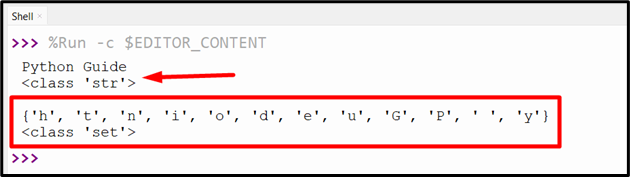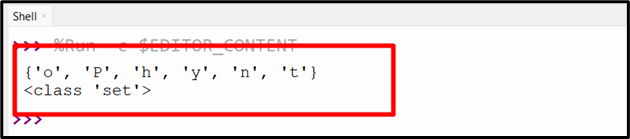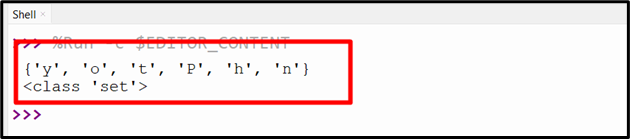| by Arround The Web | No comments

## Convert String to Set Python

Converting a string to a set in Python assists in easier manipulation of the text, as it removes duplicate characters and orders the characters in the string alphabetically. Python provides various ways to convert the input string to a set. In this post, we will overview each of these methods using the appropriate examples.

This article will cover the following approaches to convert the specified strings to set in Python:

## Method 1: Convert String to Set in Python Using the “set()” Function

The “set()” function creates the Python set object which has unique and unordered elements. The method can be used here to perform the discussed conversion.

Syntax

set(iterable)

Here, “iterable” is an optional parameter that specifies the “sequence”, “collection”, or “iterator object” that needs to be converted into a set. If an iterable is not given, an empty set is created.

Example

Let’s overview the following example code:

string_value = "Python Guide"
print(string_value)
print(str(type(string_value)), '\n')
set_value = set(string_value)
print(set_value)
print(str(type(set_value)))

In the above code, the “string” value is initialized and converted into the set using the “set()” function. The “type()” function is applied twice before and after the conversion(to set) to check the data type.

OutputThe “string” in the above snippet has been converted into a “set“.

## Method 2: Convert String to Set in Python Using the “add()” Method

In Python, the “add()” method is utilized to add an element/item to a set. The “add()” method takes a single argument which is the element/items to be added to the set. It does not return any value. This method can be applied to append the initialized string into an empty set.

Syntax

In the above syntax, “set” is the name of the set object, and “element” corresponds to the item that needs to be added.

Example

The below example code is used to convert string to set in Python:

string_value = "Python"
set_value = set()
for character in string_value:
print(set_value)
print(type(set_value))

In the above lines of code, the string, and empty set are initialized, respectively. After that, the “for” loop is applied along with the “add()” function to iterate over the string and append the string characters into the empty set.

OutputThe above output shows that the string has been iterated and appended into the set accordingly.

## Method 3: Convert String to Set in Python Using “Set Comprehension”

In Python, “set comprehension” is utilized to generate a set from a current iterable, such as a list or a string. This approach is utilized to convert the given string into the set via a “for” loop.

Syntax

{expression for element in iterable if condition}

In the above syntax:

• expression” is any valid Python expression that can be applied to the element.
• iterable” is any iterable object like a set, tuple, list, etc.
• condition” is an optional filter that selects which elements to include in the new set.

Example

Let’s overview the following code:

string_value = "Python"
set_value = {i for i in string_value}
print(set_value)
print(type(set_value))

In the above code block, “set comprehension” is used along with the “for” loop to create a set with each string character as individual elements.

OutputBased on the above output, it can be verified that the input string has been converted into a set. It is such that the string characters are appended into a set.

## Conclusion

To convert the given string to a set, the “set()” function, “add()” method, or “set comprehension” can be used in Python. The inbuilt “set()” function is used along with the “str()” function to transform/convert the given string to a set in Python. The “add()” and “set comprehension” approaches perform the conversion via iterating through the string value. This Python post presented various ways to convert the string to a set.

Source: linuxhint.com